# Generality versus core insight trade-off in theorem statements

For example, consider the calculus:Intermediate value theorem . It's possible to state the theorem as saying the function attains each value between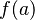$f(a)$ and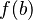$f(b)$. But this introduces an extra parameter which takes up more working memory. It is simpler to state it as saying the function equals zero somewhere. Similarly, we could let$a$ and$b$ be any real numbers, but we could restrict the theorem to the case$a < b$, which is where all of the interesting stuff happens.
for any$t$ between$f(a)$ and$f(b)$, there is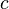$c$ in$[a,b]$ such that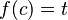$f(c) = t$ there is$c$ in$[a,b]$ such that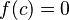$f(c) = 0$$a,b$ are arbitrary real numbers (including the case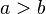$a > b$, which makes$[a,b]$ empty so the theorem becomes vacuous, and also including the case$a=b$ so the function consists of a single point, which makes the theorem trivial)$a,b$ are real numbers such that$a$f(a)$ and$f(b)$ can be anything (we don't add hypotheses concerning their values)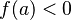$f(a) < 0$ and$f(b) > 0$